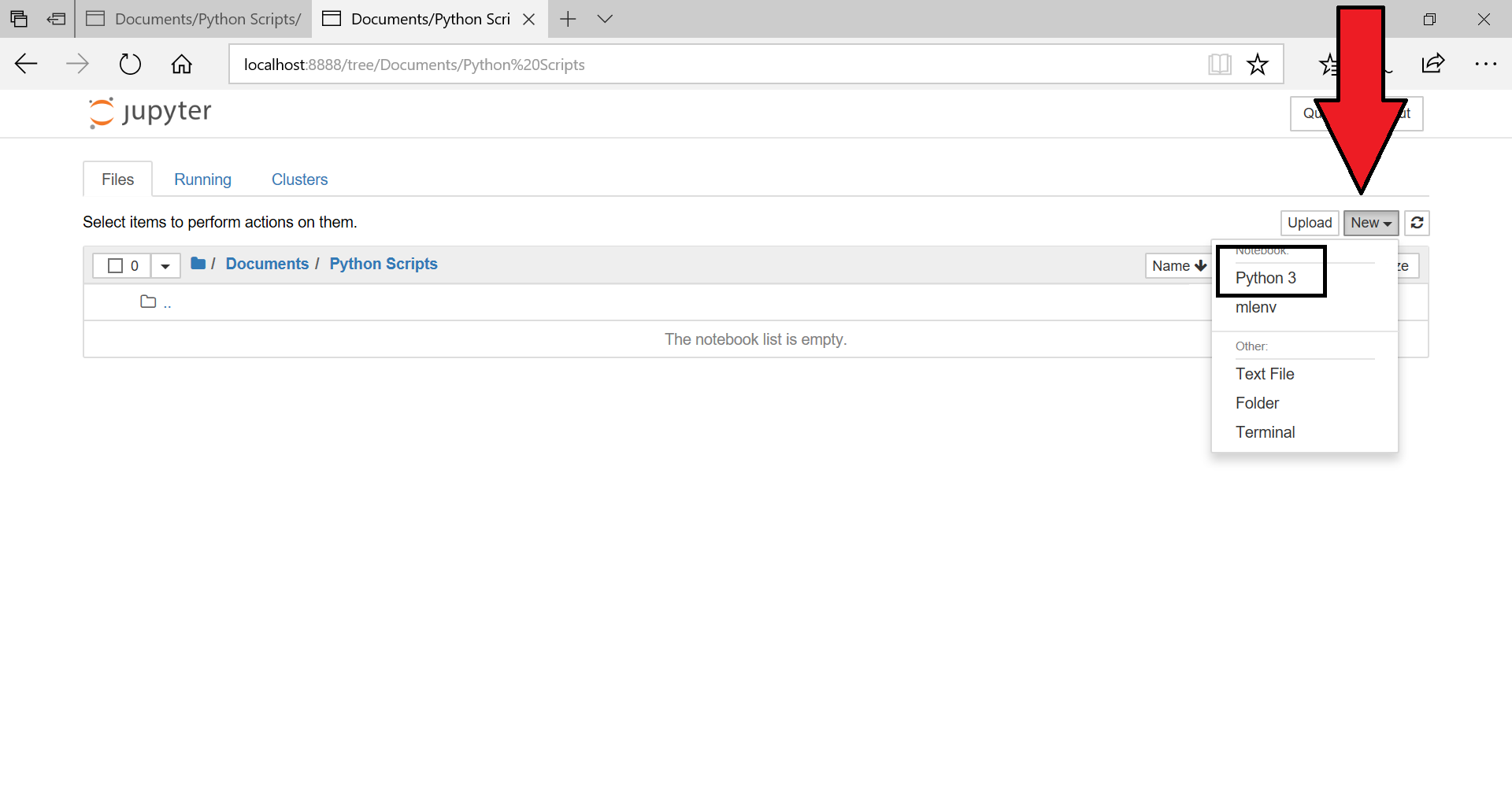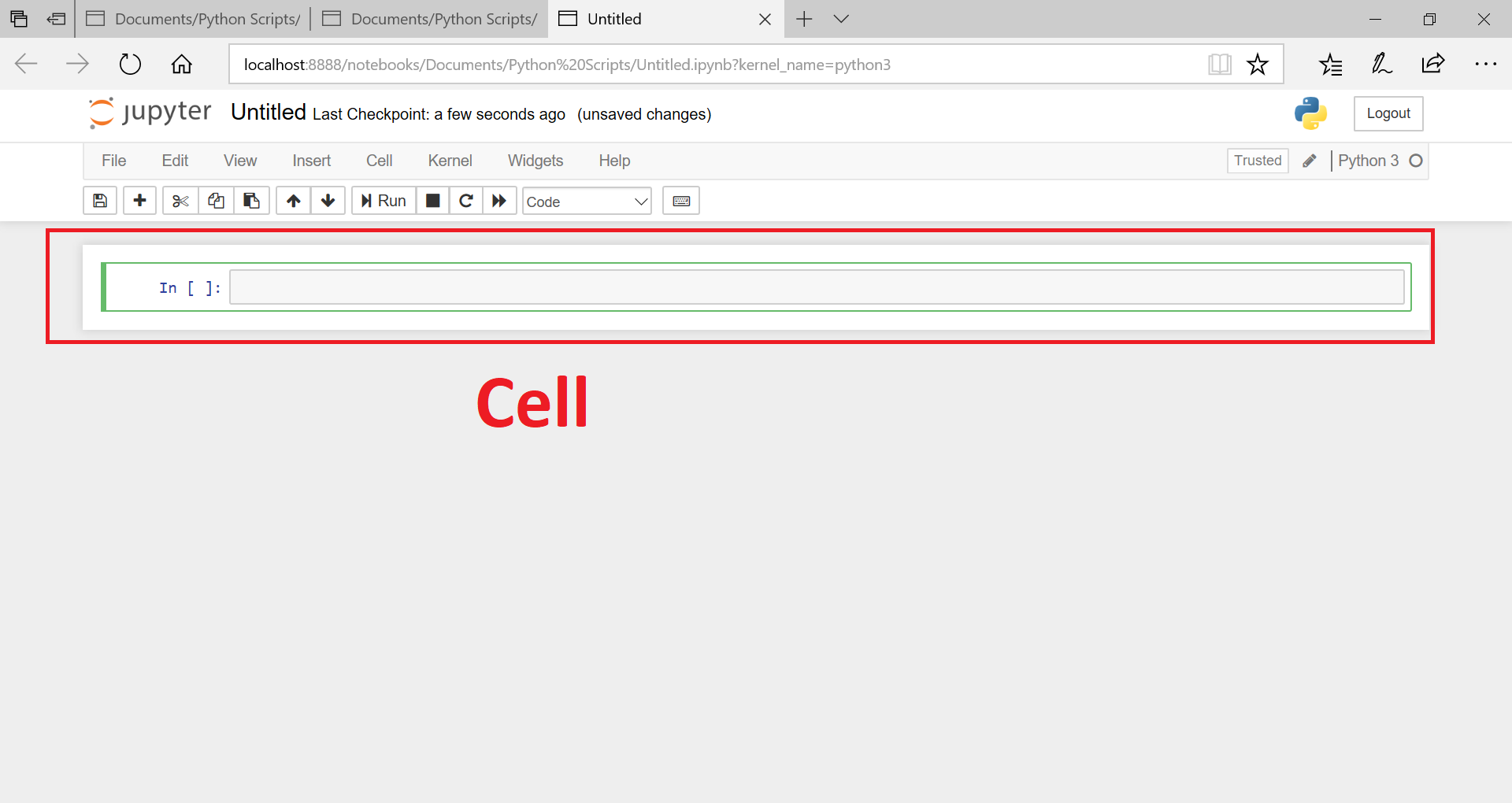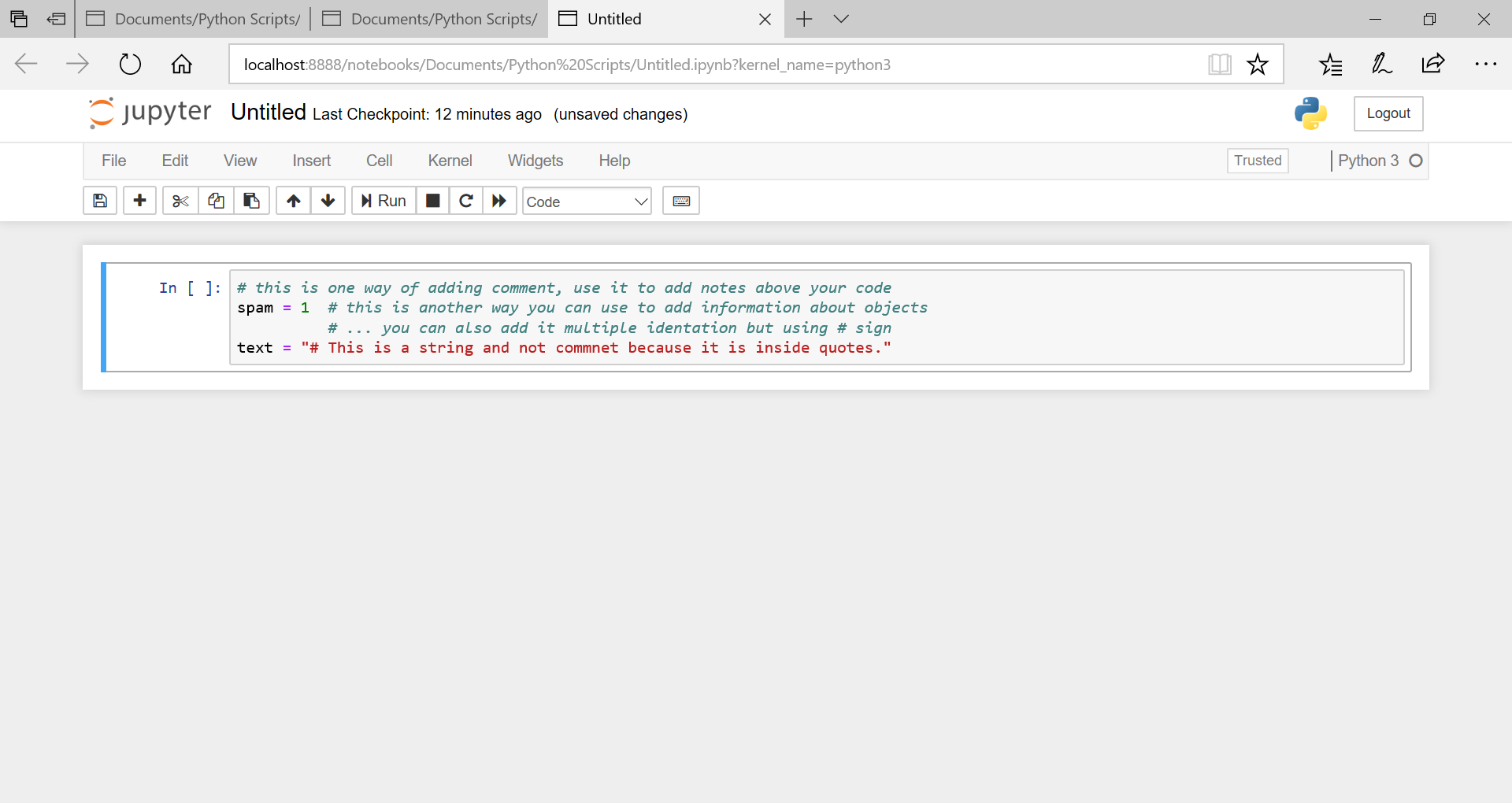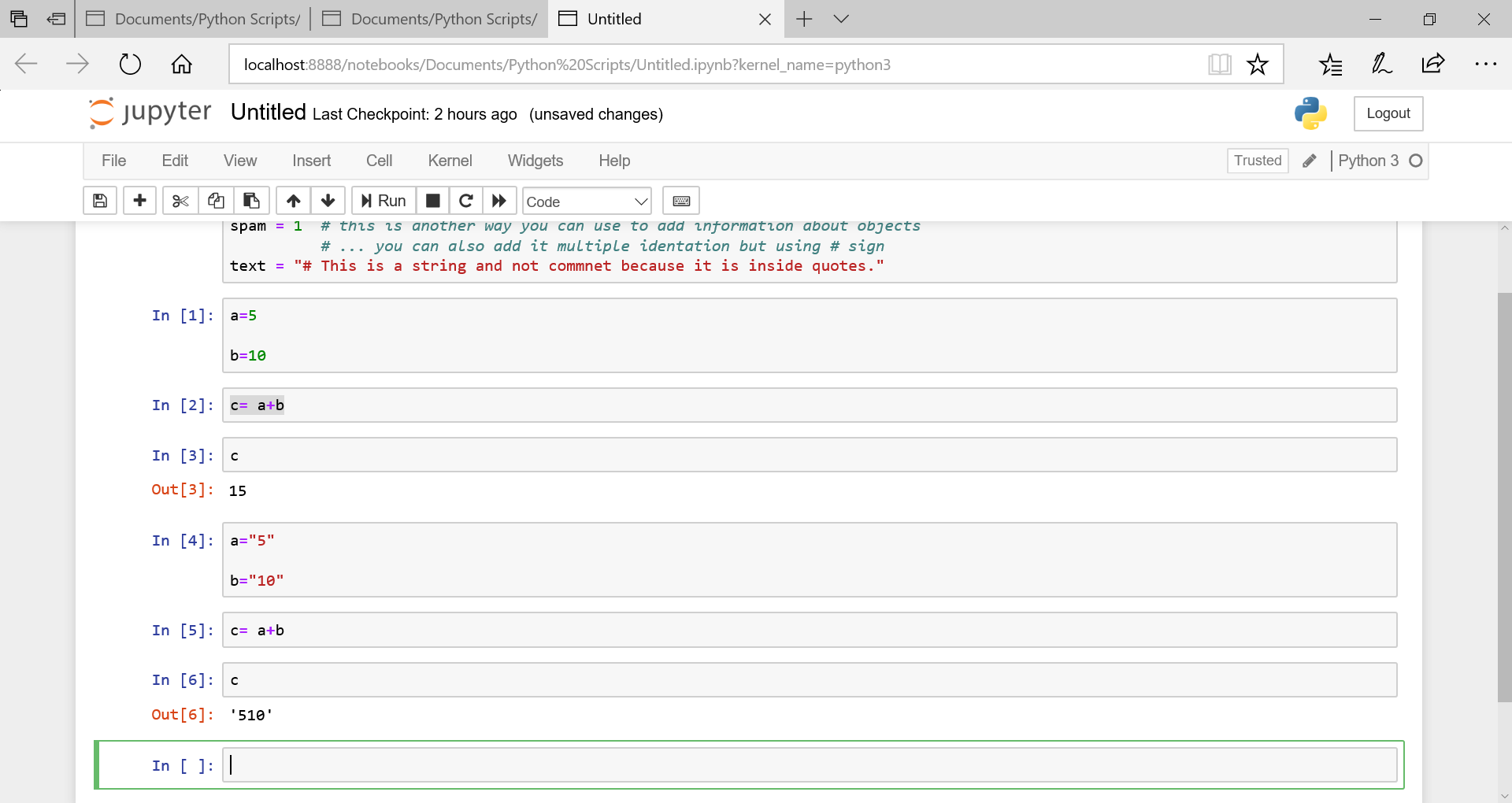# Python Tutorial For Beginners-Comments & Variables

### Machine learning & Data science

Complete machine learning & data science course to help you get into one of the hottest jobs on the planet.

In this python tutorial for beginners, we are going to cover fundamentals of python programming language. if you are yet to install python & jupyter notebook on your machine, please click here to go back and install them.

In this post, i am going to cover overview of python language and in the next topic, i will talk about python data types which are core to machine learning & data science.

Please note these posts are intended to act as an enabler for you to learn machine learning & data science. They are not very exhaustive as far as python is concerned.

If you are looking to use python for some other purpose like web development, these will help you with the basics but you will have to learn advance python to work in those fields.

In machine learning, python is used with libraries like numpy, pandas, scikit-learn, tensorflow & keras. And these libraries do most of the heavy lifting therefore i will be focusing on them.

Python language was invented by Guido van Rossum. Language itself is over two decades old but the kind of popularity and momentum it has gained in last 5 years, is simply incredible.

# Python Tutorial for Beginners

## Python Overview

Python is object oriented, dynamically typed and strongly typed programming language.

As mentioned in last chapter, we are going to use Python 3 for the syllabus because Python 2.X is legacy and Python 3.X is the default version for python now and all new projects should use Python 3 only.

You have already seen the basic syntax of Python which is;

print("hello world")

Point to be noticed- Python is a case sensitive language therefore hello world is not equal to HELLO WORLD or Hello World.

You can use single or double quotes with Python 3.

You don't need to use quotes when printing numbers, for example;

print(2) will print 2 in the console.

And yes, don't bother about "print", it is a python function which is simply producing output here. We will talk about functions later. For now, print will produce whatever input you will provide inside the parenthesis/brackets.

If you are coming from some other language like PHP, then you need to get rid of your habit of putting semi-colon at the end of each command, you don't need that with Python.

So, let’s continue with our Python overview. Assuming you completed all the steps mentioned in last post, you have jupyter notebook up and running. When you create a notebook, it is created with a blank row which is called “Cell”.You can spend some time with the top menu to understand Jupyter notebook. It is quite simple and won’t take much effort to learn so I am not putting more effort in this direction. I will guide you when situation arises based on our requirement.

We will use these cells to run Python code in it. They follow Python syntax, so you can easily use them to add comment just like you will use in any other editor. So, feel free to experiment with it. And if you don’t know how to add comments in python, you can do that by using # (hash) sign.

Python comments are again very simple and like other programming languages. You just need to "#" sign before a single line comment for example;

# this is a sample comment and interpreter will not run it

Multiple Line Comment in python

'''

Your

sample

comment

'''

Here are some of the examples;## Python Variables

Let’s start with something which is simpler, math. Now I know you would think “MATH!!!”.

Before you get mad at me for using math, let me clarify, I am taking about simple math and as this book is about learning data science, it will help us in getting our feet wet.

We will use basic math to learn and understand python variables.

Variables are integral to any programming language. If you are coming from math's background or any other programming language, you know pretty much about it.

But for those who are not aware of the concept of variables, next few lines will help you in understanding the concept of variables in a programming language. Variables are integral to any programming language. If you are coming from math's background or any other programming language, you know pretty much about it.

But for those who are not aware of the concept of variables, next few lines will help you in understanding the concept of variables in a programming language.

### Variables in programming

This concept of variables is not exclusive to python and broadly applies to most of the programming languages.

Concept of variables are rooted in the math and further extended in most programming languages.

Variables are used to store values in Python, or for that matter, any other language.

When you know that the value of an entity may change overtime (real time changes or at fixed intervals/triggers) you can't use a fix value for it, you will use a variable and assign a value to it.

Variables can be updated real time to make sure that program is reflecting the correct/updated values.

Example-In algebra, you use variable to calculate the value (because you may receive any parameters), something like;

area= length * width * height

now, as mentioned earlier, you may receive any value for the above-mentioned parameters like length, width and height. You simply replace above parameters with the received value something like;

area= length * width * height

4               4            4        (I have used 4 as example, you can use any value)

As it is following a formula, it will produce output based on the input values following same formula each time. So, formula and variables will remain same but with different values of variables, formula will produce different output.

Similarly, you use variables in programming to perform these calculations.

Now, there are four variables in the above formula;

• area
• length
• width
• height

In programming, you create variables for each value and replace it in the code.

So if you assign following values to these variables;

• a= area
• l= length
• w= width
• h=height

a= l * w * h

now if you assign values (we will use 4 for this example) to these variables in python, it will be like;

• l = 4
• w= 4
• h= 4

a will be the output of these multiplications, you just need to replace these values and your calculation will produce correct result.

Variables are thoroughly used in programming languages to assign and store values.

Some of the examples would be,

• Writing simple to complex program as shown above
• Receiving and Manipulating form inputs using variables where variables are used to assign values to input values like name etc.

### Creating Variables in Python 3

Now for those who are coming from other programming languages, please leave your comments to add more info about variables to help other. I am trying to put it in the least possible words to make sure that newbies are not getting lost in typical programming jargon.

Ok, so coming back to how to create variables in Python 3.

I have already shown how to create variables in python above.

You don't need to specify anything in python to inform it that the entity is an variable, you can simply use the name of the variable and assign value to it;

x=5

There is no need to put prefix like var before variable name or any suffix after the value. Python is smart enough to identify it as a variable and declare it as variable for you(automatically).

When you use strings in the variable, you need to use quotes and even if you put number in quotes, python will treat it as strings.

So if you have a program with two variable;

a=5

b=10

As there are no quotes in the above example, python will treat them as numbers. Now if you perform;

c= a+b

you will get 15

But if you use quotes with the above variables, python will treat them as string;

a="5"

b="10"

now if you run c=a+b, you will get;

c="510"

Because python will simply join these strings which is called concatenation.Note- Please note that when you print variables, do not use quotes with the print otherwise python will treat it as a string and not a variable which simply mean;

if you use;

print(c)

you will get value of c variable whereas when you use

print("c")

python will simply print c

This concept of treating variables as string or number is called casting.

You can control how python treats your variable by using certain python function like;

int(variable) # int stands for integer, it will cast your variable to a integer

float(variable) # as the name suggest, floating numbers

str(variable) # str stands for string which mean your variable will be treated as string

There are few other functions also which are used for casting but at this stage, let's leave it at that.

If you try to use a variable in the program without declaring it, you will get an exception error.

• Name can start with a _, capital letter or small letter
• You can use numbers in your variable name but after first letter
• You can't use name of the python functions in your variable name.

This is a very basic overview of Python variables. I don't want to add too much information here to avoid any confusion.

You can spend some time practicing with these variables. Create more and bigger variables and try to use mathematical operations on them. Also try these casting functions and see what happens.About akhilendra

1.FavTutor says
2.Samira says